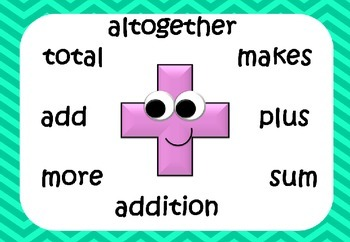# Magic of Maths

36.4K Views

Find out a multi-digit number that if multiplied by the number 9 or any of its multiplications products (i.e. 18, 27, 36, 45,..) will result in the multiplication factor repeated (n) number of times.There is no single solution that fits all. A somewhat generic form could be the N-digit number such that the number of digits equals ((number of digits of the multiple of 9) + 1), wherein the first and last digits are 1 and the intermediate digits are 0s.

e.g. When the multiplier is 9: No. of digits  of multiplier = 1, hence the required number is the two-digit (1 + 1) number with first & last digits as 1, i.e., 11. (9 x 11= 99)

When Multiplier is 18, 27… 99, (No. of digits = 2) the required number is three-digit (2 + 1) number with 1st & last digits as 1 and remaining 0., i.e,, the number 101.

101 x 18 = 1818
101 x 27 = 2727
101 x 36 = 3636 and so on.

101 x 99 = 9999.

When the  multiplier is more than 99: (i.e., 108, 117, 126…) No. of digits = 3, and the required number four-digit (3+1) number 1001.

101 x 108 = 108108
101 x 117 = 117117
101 x 126 = 126126 and so on.